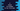# C program to check if a number is positive,negative or zero using macros### C programming to check if a number is positive, negative or zero using macros :

In this programming tutorial, we will learn how to check if a user input number is zero, negative or positive. Our program will ask the user to enter a number. Then it will check if the number is zero, positive or negative using a macro function. Let’s take a look at the program first :

### C program :

``````#include<stdio.h>

//1
#define ISZERO(number) (number == 0)
#define ISPOSITIVE(number) (number > 0)
#define ISNEGATIVE(number) (number < 0)

int main(){
int num;

//2
printf("Enter a number : ");
scanf("%d",&num);

//3
if(ISZERO(num)){
printf("It is a zero.");
}else if(ISPOSITIVE(num)){
printf("It is a positive number.");
}else if(ISNEGATIVE(num)){
printf("It is a negative number.");
}

return 0;
}
``````

### Explanation :

The commented numbers in the above program denote the step number below :

1. Create three different macros to check if the number is zero, positive or negative.
2. Ask the user to enter a number. Read it and store it in variable num.
3. Now using three if-else if conditions, check if the number is zero, positive or negative. Also, print out one message if any of these three checks is true.

### Sample Output :

``````Enter a number : 0
It is a zero.
Enter a number : 3
It is a positive number.
Enter a number : -4
It is a negative number.
``````